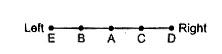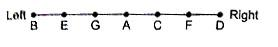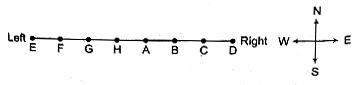## Seating Arrangement For SBI PO Set – 51

1 – 2) Read the following information carefully and then answer the questions given below.

Seven boys A, B, C, D, E, F and G are  standing in a line.

I.        G is between A and E.

II.       F and A have one boy between then.

III.     E and C have two boys between them.

IV.      D is to the immediate right of F.

V.       C and B have three boys between them.

1). Who is second from left ?

a)   C

b)   G

c)   E

d)   A

e)   None of these

2). C is standing between

a)   A and F

b) D and G

c)  A and D

d)   F and G

e) None of these

3 – 5) Read the following information carefully and then answers the questions given below it. Five friends A, B, C, D and E are sitting on a bench. A is sitting next to B.C is sitting next to D.D is not sitting with E.E is on the left end of the bench.C is on second position from right.  A is on the right side of B and to the right side of E.A and C are sitting together.

3) At what position is A sitting ?

a)   Between B and C

b)   Between D and C

c)   Between E and D

d)   Between C and E

e)   None of these

4) Who is sitting at the centre?

a)   A

b)   B

c)   C

d)   D

e)   None of these

5) What is the position of B ?

a)   Second from right

b) Centre

c)   Extreme left

d) Second from left

e)   None of these1 c 2 a 3 a 4 a 5 d

7 – 11) Read the following information and answer the questions given below

I.    A, B, C, D, E, F, G and H are sitting in a row facing North.

II.   A is fourth to the right of E.

III.  H is fourth to the left of D.

IV.  C and F, who are not at the ends, are neighbours of B and E, respectively.

V. H is next to the left of A and A is the neighbour of B.

7). What is the position of F?

a)   Next to the right of E.

b)   Next to the right of G.

c)   Sixth to the right of D. d)   Between G and H.

e)   None of these

8) . Which of the following statements is not true ?

a)   G is the neighbour of H and F b)   B is next to the right of A.

c)   E is at left end d)   D is next to the right of B

e)   None of these

9). Who is/are the neighbour/(s) of D ?

a)   F alone

b)   C alone

c)   B and C

d)   Cannot be determined

e)   None of these

10). Which of the following statements is not true ?

a)   H is second to the right of F

b)   E is fourth to the left of A

c)   D is fourth to the right of H

d)   None of these

e)   Cannot be determined

11). Who are sitting at the ends ?

a)   E and C

b)   F and D

c)   G and B

d)   None of these

e)   Cannot be determined